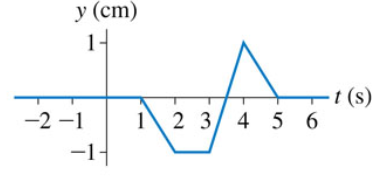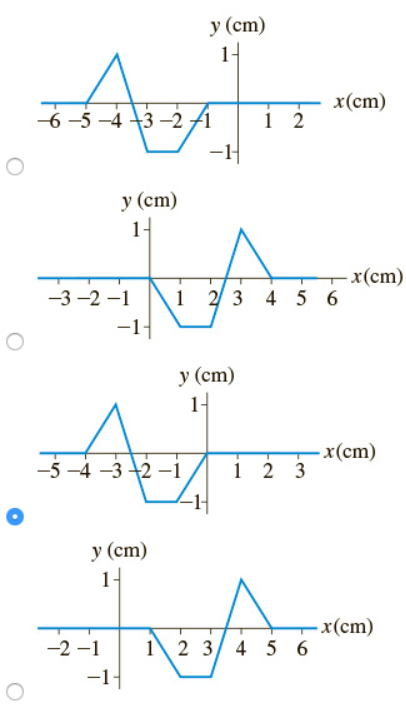# Problem: (Figure 1) shows a history graph at x = 0 cm of a wave moving to the left at 1 cm/s.What is the snapshot graph of this wave at t = 1 s?

###### FREE Expert Solution

The graph shows the velocity of the wave.

The leading edge of the wave reaches x = 0 at t = 1 s, based on the history graph for x = 0.

98% (306 ratings)###### Problem Details

(Figure 1) shows a history graph at x = 0 cm of a wave moving to the left at 1 cm/s.What is the snapshot graph of this wave at t = 1 s?